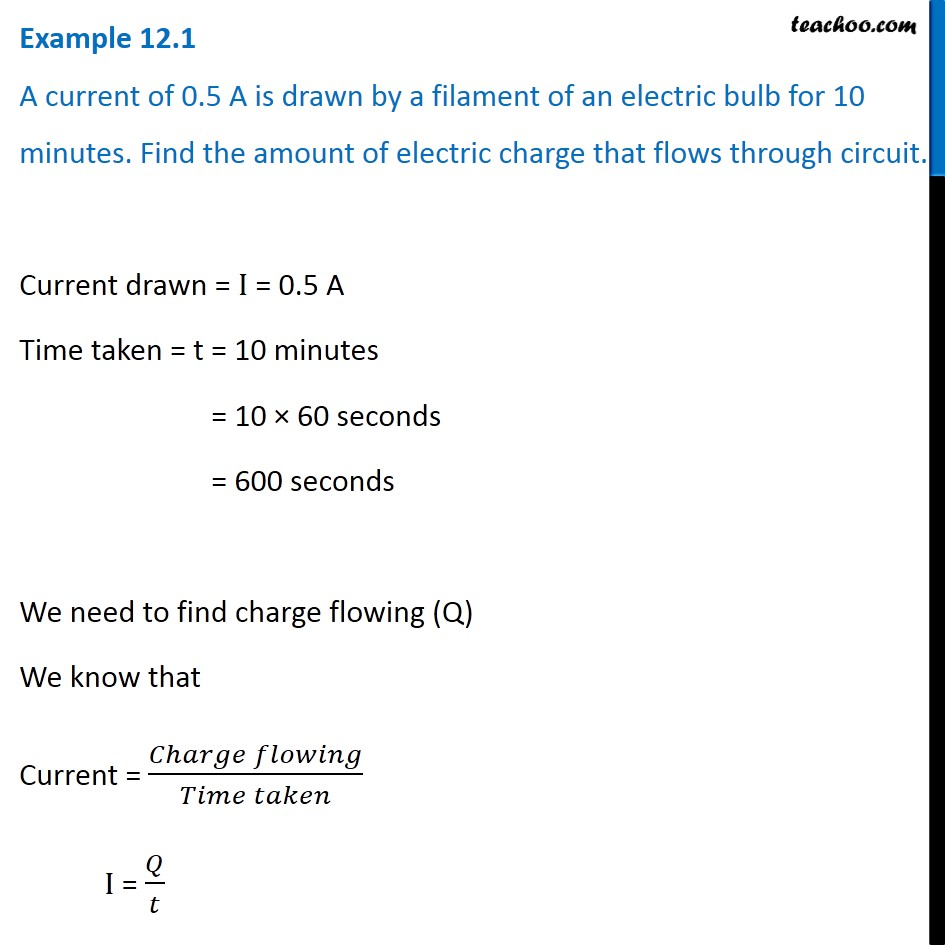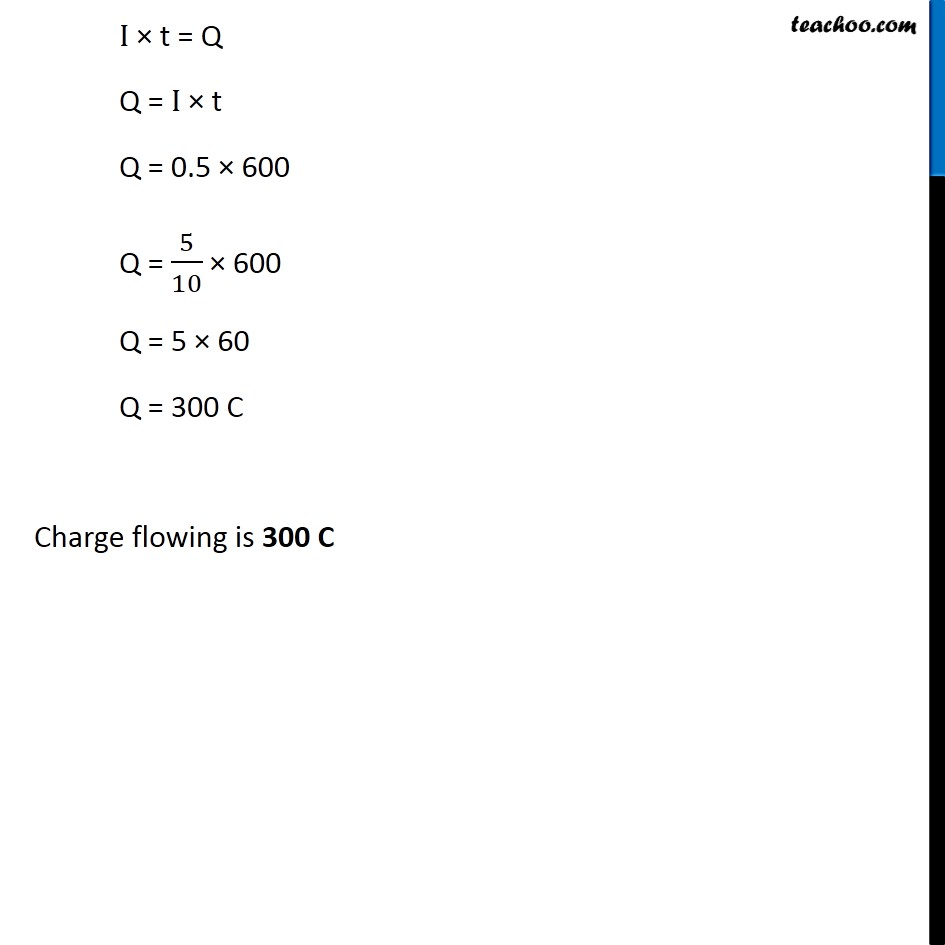Examples from NCERT Book

Class 10
Chapter 12 Class 10 - Electricity

## A current of 0.5 A is drawn by a filament of an electric bulb for 10 minutes. Find the amount of electric charge that flows through circuit.Learn in your speed, with individual attention - Teachoo Maths 1-on-1 Class

### Transcript

Example 12.1 A current of 0.5 A is drawn by a filament of an electric bulb for 10 minutes. Find the amount of electric charge that flows through circuit. Current drawn = I = 0.5 A Time taken = t = 10 minutes = 10 × 60 seconds = 600 seconds We need to find charge flowing (Q) We know that Current = (𝐶ℎ𝑎𝑟𝑔𝑒 𝑓𝑙𝑜𝑤𝑖𝑛𝑔)/(𝑇𝑖𝑚𝑒 𝑡𝑎𝑘𝑒𝑛) I = 𝑄/𝑡 I × t = Q Q = I × t Q = 0.5 × 600 Q = 5/10 × 600 Q = 5 × 60 Q = 300 C Charge flowing is 300 C